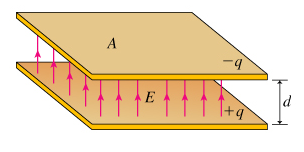# Problem: (a) Assume that charge −q is placed on the top plate, and +q is placed on the bottom plate. What is the magnitude of the electric field E between the plates? Express E in terms of q and other quantities given in the image, in addition to ε0 and any other constants needed.(b) What is the voltage V between the plates of the capacitor? Express V in terms of the quantities given in the image and any required physical constants.(c) Now find the capacitance C of the parallel-plate capacitor. Express C in terms of quantities given in the image and constants like ε0.

###### FREE Expert Solution
93% (172 ratings)View Complete Written Solution
###### Problem Details(a) Assume that charge −q is placed on the top plate, and +q is placed on the bottom plate. What is the magnitude of the electric field E between the plates? Express E in terms of q and other quantities given in the image, in addition to ε0 and any other constants needed.

(b) What is the voltage V between the plates of the capacitor? Express V in terms of the quantities given in the image and any required physical constants.

(c) Now find the capacitance C of the parallel-plate capacitor. Express C in terms of quantities given in the image and constants like ε0.